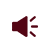• ISSN 1001-1455  CN 51-1148/O3
• EI、Scopus、CA、JST收录
• 力学类中文核心期刊
• 中国科技核心期刊、CSCD统计源期刊引用本文: 李斌, 朱志武, 李涛. 冻融循环冻土的冲击动态力学性能[J]. 爆炸与冲击.LI Bin, ZHU Zhiwu, LI Tao. Impact dynamic mechanical properties of frozen soil with freeze-thaw cycles[J]. Explosion And Shock Waves. doi: 10.11883/bzycj-2021-0475
 Citation: LI Bin, ZHU Zhiwu, LI Tao. Impact dynamic mechanical properties of frozen soil with freeze-thaw cycles[J]. Explosion And Shock Waves.## 冻融循环冻土的冲击动态力学性能

##### doi: 10.11883/bzycj-2021-0475

###### 通讯作者: 朱志武（1974－ ），男，博士，教授，zzw4455@163.com
• 中图分类号: O347.3

## Impact dynamic mechanical properties of frozen soil with freeze-thaw cycles

• 摘要: 以典型冻土为研究对象，通过不同冻融循环次数的冻融循环实验、不同温度的冻结实验以及不同应变率的冲击动态实验，综合研究了冻融循环冻土的冲击动态力学性能。结果表明，冻土存在冻融循环效应，随着冻融循环次数的增加，冻土的峰值应力有一定程度的降低，但在达到临界冻融循环次数后，峰值应力将维持稳定；同时，冻土表现出明显的应变率效应和温度效应，其峰值应力随应变率的增加或温度的降低而增加。通过定义冻融损伤因子，推导满足Weibull分布的冲击损伤，提出了一个基于Z-W-T方程的损伤黏弹性本构模型。该模型可较好地描述冻融循环后冻土的冲击动态力学行为，为研究季节性冻土区冻土的冲击动态破坏提供参考。
• 图  1  圆柱土体试样

Figure  1.  Cylindrical soil specimen

图  2  温度时程曲线与冻融循环实验装置

Figure  2.  2Temperature time history curve and freeze-thaw cycles experimental device

图  3  SHPB实验装置

Figure  3.  A SHPB device

图  4  典型波形图

Figure  4.  Typical waveform

图  5  不同工况下冻土的应力-应变曲线图 (T = −20 ℃)

Figure  5.  Stress-strain curves of frozen soil for different cases (T = −20 °C)

图  6  不同工况下冻土的应力-应变曲线图 ($\dot \varepsilon$= 550 s−1)

Figure  6.  Stress-strain curves of frozen soil for different cases ($\dot \varepsilon$= 550 s−1)

图  7  不同工况下冻土的冻土峰值应力

Figure  7.  Peak stress of frozen soil for different cases

图  8  冻结过程示意图

Figure  8.  Schematic diagram of the freezing process

图  9  冻融损伤因子

Figure  9.  Freeze-thaw damage factor

图  10  Z-W-T本构模型

Figure  10.  Z-W-T constitutive model

图  11  相同温度不同应变率下冻土的理论曲线与实验曲线(T = −20 ℃)

Figure  11.  Theoretical and experimental curves of frozensoil at the same temperature and different strain rates(T = −20 °C)

图  12  相同应变率不同温度下冻土的理论曲线与实验曲线($\dot \varepsilon$= 550 s−1)

Figure  12.  Theoretical and experimental curves of at the same strain rate and different temperatures ($\dot \varepsilon$= 550 s−1)

表  1  实验方案

Table  1.   Experimental scheme

 冻融循环次数 T/℃ $\dot \varepsilon {\text{/}}{{\text{s}}^{{\text{−1}}}}$ 0 −20 550，450，350 −15 550 −10 550 1 −20 550，450，350 −15 550 −10 550 3 −20 550，450，350 −15 550 −10 550 5 −20 550，450，350 −15 550 −10 550

表  2  冻融循环冻土冲击实验结果

Table  2.   Experimental results of frozen soil with freeze–thaw cycles under impact loading

 冻融循环次数 T/℃ $\dot \varepsilon {\text{/}}{{\text{s}}^{{\text{−1}}}}$ 实验1 实验2 实验3 ${\sigma _{\text{p}}}{\text{/MPa}}$ ${\varepsilon _{\text{p}}}{\text{/\% }}$ ${\sigma _{\text{p}}}{\text{/MPa}}$ ${\varepsilon _{\text{p}}}{\text{/\% }}$ ${\sigma _{\text{p}}}{\text{/MPa}}$ ${\varepsilon _{\text{p}}}{\text{/\% }}$ 0 −10 550 6.74 4.07 7.16 4.13 6.96 4.11 −15 550 8.71 4.21 8.42 4.14 8.53 3.91 −20 350 8.55 2.34 8.29 2.54 8.19 2.45 450 9.67 3.36 9.78 3.25 10.11 3.68 550 11.13 4.34 11.06 4.18 10.69 4.29 1 −10 550 6.22 4.16 5.91 4.31 6.42 4.2 −15 550 7.75 3.96 7.55 3.91 7.82 4.12 −20 350 7.48 2.39 7.64 2.58 7.51 2.44 450 8.37 3.43 8.64 3.15 8.74 3.25 550 9.61 4.1 9.51 4.13 9.81 4.07 3 −10 550 5.96 4.13 6.4 4.15 6.41 4.26 −15 550 7.41 4.19 7.95 4.24 7.11 4.11 −20 350 6.72 2.74 7.03 2.51 7.11 2.82 450 8.97 3.41 8.54 3.38 8.62 3.45 550 9.31 4.23 9.54 4.11 9.31 4.08 5 −10 550 6.15 4.32 6.32 4.23 5.92 4.22 −15 550 7.42 4.28 7.12 4.18 7.71 4.13 −20 350 7.11 2.28 7.02 2.21 7.21 2.34 450 8.54 3.08 8.61 3.03 8.54 2.94 550 9.62 4.13 9.36 4.11 9.51 3.92

表  3  本构模型参数 ($T=-20\;^{circ}{\rm C}$)

Table  3.   Constitutive model parameters ($T=-20\;^{circ}{\rm C}$)

 冻融循环次数 $\dot \varepsilon {\text{/}}{{\text{s}}^{{\text{−1}}}}$ ${E_{\text{0}}}{\text{/GPa}}$ ${E_2}{\text{/GPa}}$ ${\theta _2}{{/\mu {\rm{s}}}}$ ${\varepsilon _{\text{f}}}$ $m$ $f$ 0 550 1.636 11.23 0.705 0.0131 1.16 1.000 450 1.667 7.36 0.971 0.0116 1.23 1.000 350 1.606 4.19 2.863 0.0088 1.23 1.000 1 550 1.655 9.16 0.671 0.0129 1.33 0.871 450 1.560 8.63 0.919 0.0114 1.32 0.871 350 1.624 4.45 2.721 0.0086 1.33 0.871 3 550 1.732 10.23 0.513 0.0139 1.13 0.847 450 1.630 13.21 0.541 0.0122 1.21 0.847 350 1.652 13.52 0.779 0.0091 1.11 0.847 5 550 1.648 14.51 0.542 0.0137 1.14 0.852 450 1.625 11.01 0.467 0.0119 1.07 0.852 350 1.626 14.06 0.467 0.0091 1.17 0.852

表  4  本构模型参数 ($\dot \varepsilon = 550\;{\rm s}^{-1}$)

Table  4.   Constitutive model parameters ($\dot \varepsilon=550\;{\rm s}^{-1}$)

 冻融循环次数 T/℃ ${E_{\text{0}}}{\text{/GPa}}$ ${E_2}{\text{/GPa}}$ ${\theta _2}{{/\mu {\rm{s}}}}$ ${\varepsilon _{\text{f}}}$ $m$ $f$ 0 −20 1.636 11.23 0.705 0.0131 1.16 1.000 −15 1.522 7.25 0.577 0.0134 1.03 1.000 −10 1.340 13.22 0.127 0.0131 1.02 1.000 1 −20 1.655 9.16 0.671 0.0129 1.34 0.871 −15 1.531 16.12 0.209 0.0129 1.12 0.888 −10 1.335 9.01 0.151 0.0131 1.05 0.939 3 −20 1.732 10.23 0.512 0.0139 1.13 0.847 −15 1.541 10.39 0.257 0.0134 1.14 0.881 −10 1.381 4.07 0.397 0.0134 1.02 0.893 5 −20 1.648 14.5 0.542 0.0137 1.14 0.852 −15 1.455 8.86 0.623 0.0134 1.01 0.875 −10 1.153 10.83 0.411 0.0131 1.06 0.878
•点击查看大图
##### 计量
• 文章访问数:  123
• HTML全文浏览量:  16
• PDF下载量:  54
• 被引次数: 0
##### 出版历程
• 收稿日期:  2021-11-15
• 修回日期:  2022-04-25
• 网络出版日期:  2022-05-18

### 目录/下载:  全尺寸图片 幻灯片
• 分享
• 用微信扫码二维码

分享至好友和朋友圈